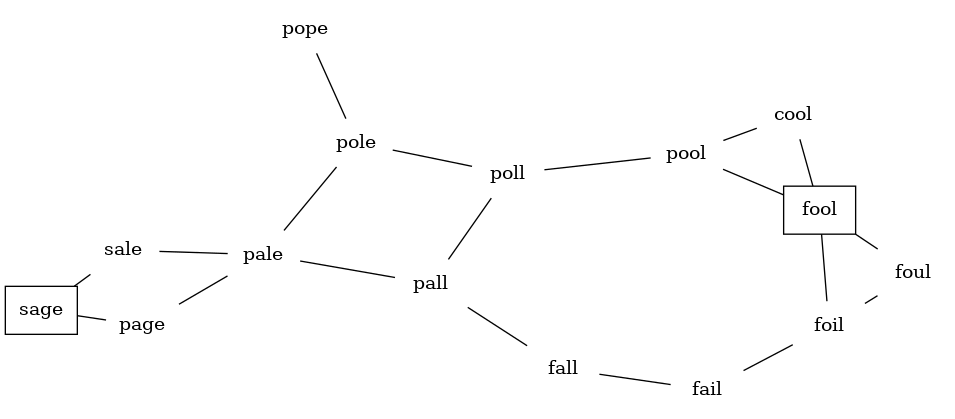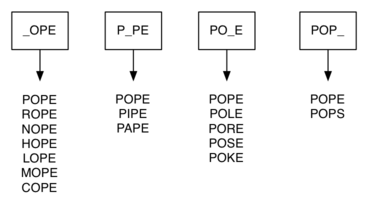# 8.8. Building the Word Ladder Graph¶

Our first problem is to figure out how to turn a large collection of words into a graph. What we would like is to have an edge from one word to another if the two words are only different by a single letter. If we can create such a graph, then any path from one word to another is a solution to the word ladder puzzle. Figure 1 shows a small graph of some words that solve the FOOL to SAGE word ladder problem. Notice that the graph is an undirected graph and that the edges are unweighted.Figure 1: A Small Word Ladder Graph

We could use several different approaches to create the graph we need to solve this problem. Let’s start with the assumption that we have a list of words that are all the same length. As a starting point, we can create a vertex in the graph for every word in the list. To figure out how to connect the words, we could compare each word in the list with every other. When we compare we are looking to see how many letters are different. If the two words in question are different by only one letter, we can create an edge between them in the graph. For a small set of words that approach would work fine; however let’s suppose we have a list of 5,110 words. Roughly speaking, comparing one word to every other word on the list is an $$O(n^2)$$ algorithm. For 5,110 words, $$n^2$$ is more than 26 million comparisons.

We can do much better by using the following approach. Suppose that we have a huge number of buckets, each of them with a four-letter word on the outside, except that one of the letters in the label has been replaced by an underscore. For example, consider Figure 2, we might have a bucket labeled “pop_.” As we process each word in our list we compare the word with each bucket, using the ‘_’ as a wildcard, so both “pope” and “pops” would match “pop_.” Every time we find a matching bucket, we put our word in that bucket. Once we have all the words in the appropriate buckets we know that all the words in the bucket must be connected.Figure 2: Word Buckets for Words That are Different by One Letter

In Python, we can implement the scheme we have just described by using a dictionary. The labels on the buckets we have just described are the keys in our dictionary. The value stored for that key is a list of words. Once we have the dictionary built we can create the graph. We start our graph by creating a vertex for each word in the graph. Then we create edges between all the vertices we find for words found under the same key in the dictionary. Listing 1 shows the Python code required to build the graph.

Listing 1

from pythonds.graphs import Graph

def buildGraph(wordFile):
d = {}
g = Graph()
wfile = open(wordFile,'r')
# create buckets of words that differ by one letter
for line in wfile:
word = line[:-1]
for i in range(len(word)):
bucket = word[:i] + '_' + word[i+1:]
if bucket in d:
d[bucket].append(word)
else:
d[bucket] = [word]
# add vertices and edges for words in the same bucket
for bucket in d.keys():
for word1 in d[bucket]:
for word2 in d[bucket]:
if word1 != word2:

Since this is our first real-world graph problem, you might be wondering how sparse is the graph? The list of four-letter words we have for this problem is 5,110 words long. If we were to use an adjacency matrix, the matrix would have 5,110 * 5,110 = 26,112,100 cells. The graph constructed by the buildGraph function has exactly 53,286 edges, so the matrix would have only 0.20% of the cells filled! That is a very sparse matrix indeed.